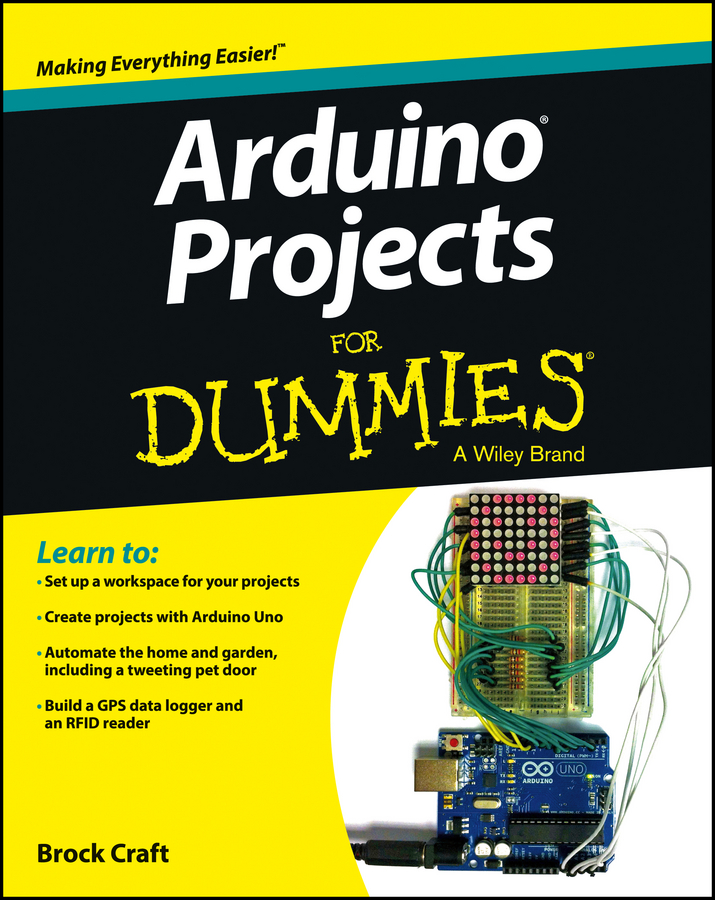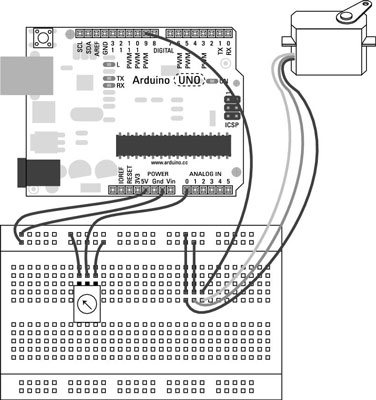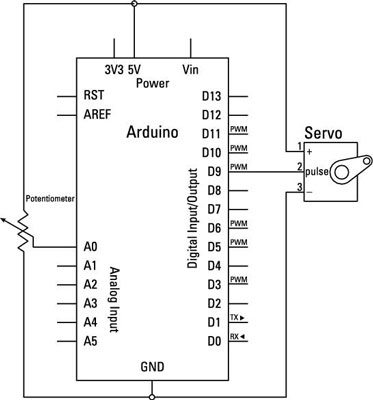##### Arduino Projects For DummiesBy using a potentiometer (or any analog sensor), it’s possible to directly control your servo with the Arduino in the same way that you’d control a mechanical claw at the arcades.

## The Knob sketch

This example shows you how you can easily use a potentiometer to move your servo to a specific degree.

You need:

• An Arduino Uno

• A servo

• A 10k ohm variable resistor

• Jump wires

The servo is wired exactly as in the Sweep example, but this time you need extra connections to 5V and GND for the potentiometer, so you must use a breadboard to provide the extra pins. Connect the 5V and GND pins on the Arduino to the positive (+) and negative (-) rows on the breadboard.

Connect the servo to the breadboard using either a row of three header pins or three jump wires. Connect the red socket to the 5V row, the black/brown socket to the GND row, and the white/yellow socket to pin 9 on the Arduino.Find a space on the breadboard for the potentiometer. Connect the center pin to pin A0 on the Arduino and the remaining pins to 5V on one side and GND on the other.After you have built the circuit, open the sketch by choosing File→Examples→Servo→Knob. The code for the sketch is as follows:

```// Controlling a servo position using a potentiometer (variable resistor)
// by Michal Rinott <http://people.interaction-ivrea.it/m.rinott>
#include <<b>Servo</b>.h>
<b>Servo</b> myservo; // create servo object to control a servo
<b>int</b> potpin = 0; // analog pin used to connect the potentiometer
<b>int</b> val; // variable to read the value from the analog pin
void setup()
{
myservo.<b>attach</b>(9); // attaches the servo on pin 9 to the servo object
}
void loop()
{
val = <b>analogRead</b>(potpin);   // reads the value of the
// potentiometer (value between
// 0 and 1023)
val = <b>map</b>(val, 0, 1023, 0, 179);  // scale it to use it with
// the servo (value between 0 and
// 180)
myservo.<b>write</b>(val);     // sets the servo position according
// to the scaled value
<b>delay</b>(15);       // waits for the servo to get there
}```

You may notice that there are a few discrepancies between the comments and the code. When referring to the range of degrees to move the servo, the sketch mentions both 0 to 179 and 0 to 180. With all Arduino tutorials, it’s best to assume that they’re works in progress and may not always be accurate.

The correct range is 0 to 179, which gives you 180 values. Counting from zero is referred to as zero indexing and is a common occurrence in Arduino, as you may have noticed by this point.

After you have found the sketch, press the Compile button to check the code. The compiler should highlight any syntax errors in the message box, which lights up red when they are discovered.

If the sketch compiles correctly, click Upload to upload the sketch to your board. When it is done uploading, your servo should turn as you turn your potentiometer.

If that isn’t what happens, you should double check your wiring:

• Make sure that you’re using pin 9 to connect the data (white/yellow) line to the servo.

• Check your connections to the potentiometer and make sure that the center pin is connected to Analog pin 0.

• Check the connections on the breadboard. If the jump wires or components are not connected using the correct rows in the breadboard, they will not work.

## The Knob sketch breakdown

In the declarations, the servo library, Servo.h, and a new servo object are named. The analog input pin is declared with a value of 0, showing that you are using Analog 0.

You may have noticed that the pin is numbered as 0, not A0 as in other examples. Either is fine, because A0 is just an alias of 0, as A1 is of 1, and so on. Using A0 is good for clarity, but optional.

There is one last variable to store the value of the reading, which will become the output.

```#include <<b>Servo</b>.h>
<b>Servo</b> myservo; // create servo object to control a servo
<b>int</b> potpin = 0; // analog pin used to connect the potentiometer
<b>int</b> val; // variable to read the value from the analog pin```

In setup, the only item to define is myservo, which is using pin 9.

```void setup()
{
myservo.<b>attach</b>(9); // attaches the servo on pin 9 to the servo object
}```

Rather than use two separate variables for input and output, this sketch simply uses one. First, val is used to store the raw sensor data, a value from 0 to 1023. This value is then processed using the map function to scale its range to that of the servo: 0 to 179.

This value is then written to the servo using myservo.write. There is also a 15 millisecond delay to reach that location. Then the loop repeats and updates its position as necessary.

```void loop()
{
val = <b>analogRead</b>(potpin);   // reads the value of the potentiometer
// (value between 0 and 1023)
val = <b>map</b>(val, 0, 1023, 0, 179);  // scale it to use it with the servo
// (value between 0 and 180)
myservo.<b>write</b>(val);     // sets the servo position according to
// the scaled value
<b>delay</b>(15);       // waits for the servo to get there
}```

With this simple addition to the circuit, it’s possible to control a servo with any sort of input. In this example, the code uses an analog input, but with a few changes it could just as easily use a digital input.